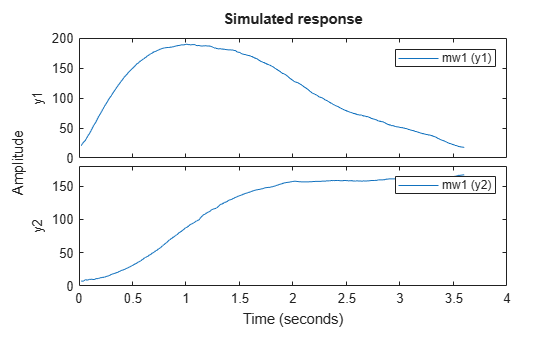# data2state

Map past data to states of state-space and nonlinear ARX models

## Syntax

``X = data2state(sys,PastData)``
``````[X,XCov] = data2state(sys,PastData)``````

## Description

example

````X = data2state(sys,PastData)` maps the past data to the states of a state-space or a nonlinear ARX model `sys`. `X` contains the state values at the time instant immediately after the most recent data sample in `PastData`. The software computes the state estimates by minimizing the 1-step ahead prediction error between predicted response and output signal in `PastData`.`data2state` is useful for continued model simulation. That is, suppose you have simulated a model up to a certain time instant and would like to then simulate the model for future inputs. Use `data2state` to estimate states of the model at the beginning of the second simulation.```

example

``````[X,XCov] = data2state(sys,PastData)``` returns the estimated covariance, `XCov`, of the current states.```

## Examples

collapse all

Compute the mapped states of an identified model, and use the states as initial state values for model simulation.

`load iddata3 z3`

Estimate a second-order state-space model using the data.

`sys = ssest(z3,2);`

Simulate the model using the entire input signal in `z3`.

```Input = z3(:,[],:); % |iddata| object containing only the input signal y_all = sim(sys,Input);```

`sim` uses zero initial conditions to compute `y_all`.

Now simulate the model using only the first-half of the input signal.

```Input1 = Input(1:150); y_1 = sim(sys,Input1);```

Continue the simulation with the second-half of the input signal such that the results show no discontinuity owing to initial-condition-induced transients. To do so, first construct a past data set comprising of the input and simulated output from the first-half of the input signal. Then calculate the state values corresponding to the start of the second-half of the input signal (t = 151).

```PastData = [y_1,Input1]; X = data2state(sys,PastData);```

`X` contains the state values at the time instant immediately after the most recent data sample in `PastData`. This time point is also the start of the future data (second-half of the input signal).

`FutureData = Input(151:end);`

Simulate the model using the second-half of the input signal and `X` as initial conditions.

```opt = simOptions('InitialCondition',X); y_2 = sim(sys,FutureData,opt);```

Verify that `y_2` matches the second half of `y_all`.

```plot(y_all,y_2,'r.') legend('Simulation using all input data',... 'Separate simulation of second-half of input data')``````load iddata1 z1 PastData = z1;```

Estimate an ARX model.

`sys = arx(PastData,[1 1 0]);`

Convert the model to a state-space model.

`sys2 = idss(sys);`

Calculate the mapped states and covariance of states using `PastData`.

`[X,XCov] = data2state(sys2,PastData);`

`X` is the state value at the time instant immediately after the most recent data sample in `PastData`.

```load motorizedcamera; z = iddata(y,u,0.02,'Name','Motorized Camera','TimeUnit','s');```

Estimate a nonlinear ARX model.

`mw1 = nlarx(z,[ones(2,2),ones(2,6),ones(2,6)],'idWaveletNetwork');`

The estimated model has six inputs and two outputs.

Determine the model order, `nx`.

`nx = order(mw1);`

Use the first `nx` samples of data to generate initial conditions.

`PastData = struct('Input', z.u(1:nx,:),'Output',z.y(1:nx,:));`

Compute the mapped states of the model.

`X = data2state(mw1,PastData);`

`X` is the state value at the time instant immediately after the most recent data sample in `PastData`.

Simulate the model using the remaining input data, and specify the initial conditions for simulation.

```InputSignal = z.u(nx+1:end,:); opt = simOptions; opt.InitialCondition = X; sim(mw1,InputSignal,opt)```## Input Arguments

collapse all

Identified model whose current states are estimated, specified as one of the following:

Past input-output data, specified as one of the following:

• `iddata` object — The number of samples must be greater than or equal to the model order. To determine model order, use `order`.

`X` is the value of model states at time ```PastData.SamplingInstants(end) + PastData.Ts```.

When `sys` is continuous-time, specify `PastData` as an `iddata` object. `X` then corresponds to the discretized (`c2d`) version of the model, where the discretization method is stored in the `InterSample`property of `PastData`.

• Structure — Specified as a structure with the following fields:

• Input — Past input data, specified as an N-by-Nu matrix, where N is great than or equal to the model order.

• Output — Past output data, specified as an N-by-Ny matrix, where N is great than or equal to the model order.

Specify `PastData` as a structure only when `sys` is a discrete-time model.

The data samples in `PastData` should be in the order of increasing time. That is, the last row in `PastData` should correspond to the latest time.

## Output Arguments

collapse all

Mapped states of model, returned as a row vector of size equal to the number of states. `X` contains the state value at the time instant immediately after the most recent data sample in `PastData`. That is, if `PastData` is an `iddata` object, `X` is the state value at time `t = PastData.SamplingInstants(end)+PastData.Ts`.

Estimated covariance of state values, returned as a matrix of size Nx-by-Nx, where Nx is the number of states.

`XCov` is empty if `sys` is a nonlinear ARX model.